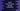# Learn to iterate through the key-value pairs of a swift dictionary## How to iterate through the key value pairs of a swift dictionary:

In this swift tutorial, we will learn how to iterate through the elements of a dictionary. Dictionary elements are key-value pairs. Using a key, we can read or modify a value.

The easiest way to loop through a swift dictionary is by using a for-in loop. We also have other ways to loop a dictionary that I am discussing below. One thing you can note that swift dictionaries are not ordered. When we iterate, there is no guarantee that the order will be the same as you have initialized it. This is mainly because dictionaries are used not to keep the order of elements. It is like a hashtable i.e. we can access the values using the keys.

## Example 1: Using a for-in loop :

Let’s consider the below example :

``````let my_dict = ["key_one": 1, "key_two": 2, "key_three": 3, "key_four": 4]

for (k,v) in my_dict{
print("key \(k), value \(v) ")
}``````

Here, we are using one for-in loop to iterate through the key, values. If you run it, it will print the below output :

``````key key_two value 2
key key_three value 3
key key_one value 1
key key_four value 4``````

## Example 2: Using forEach :

``````let my_dict = ["key_one": 1, "key_two": 2, "key_three": 3, "key_four": 4]

my_dict.forEach{print("key: \(\$0.key), value: \(\$0.value)")}``````

The output is same.

## Example 3: Using enumerated:

enumerated() returns a sequence of index and key-value pairs. For each index, there will be a key-value pair. For example :

``````let my_dict = ["key_one": 1, "key_two": 2, "key_three": 3, "key_four": 4]

for (i,v) in my_dict.enumerated(){
print("Index:\(i), value: \(v)")
}``````

It will print :

``````Index:0, value: (key: "key_four", value: 4)
Index:1, value: (key: "key_three", value: 3)
Index:2, value: (key: "key_one", value: 1)
Index:3, value: (key: "key_two", value: 2)``````